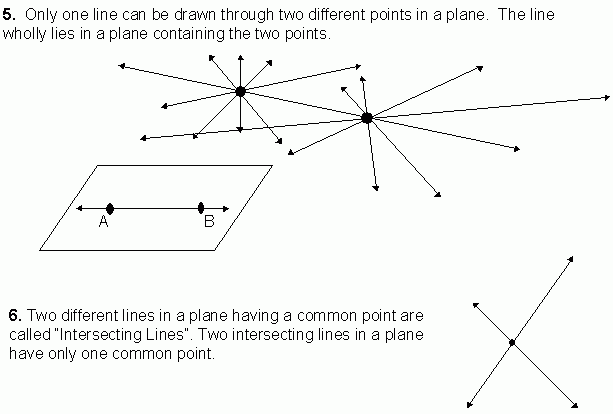Email us to get an instant 20% discount on highly effective K-12 Math & English kwizNET Programs!

#### Online Quiz (WorksheetABCD)

Questions Per Quiz = 2 4 6 8 10

### MEAP Preparation - Grade 7 Mathematics4.29 Basic Geometrical Concepts - Parallel Lines, Collinear Points and PlaneFor videos and additional resources on this topic, please see: MyndBook.com/go.php?to=207_885 Directions: Answer the following questions. Also on a sheet of paper draw a collinear points, non-collinear points and parallel and intersecting lines.
 Q 1: A plane is a flat surface extending _____ in all direction.definitelyindefinitelyfinitely Q 2: A plane is a set of _______.planespointslines Q 3: Six points are on a paper such that not any three of them are collinear. Then number of lines that can be drawn joining two points at a time is _____.51510 Q 4: The surface of a box, the cover page of a book etc., are examples of parts of _____.linesplanespoints Q 5: Number of lines that can be drawn through collinear points is _____.twooneinfinity Q 6: 'A' and 'B' are two points on a paper. If a line is passing through 'A' and 'B', then the number of other lines that can be drawn through 'A' and 'B' is _____.twozeromanyone Q 7: The flat surface of a white board gives an idea of a _____.planelinepoint Q 8: Number of lines that can be drawn through three non-collinear points, taking two points at a time is _____.onetwothree Question 9: This question is available to subscribers only! Question 10: This question is available to subscribers only!